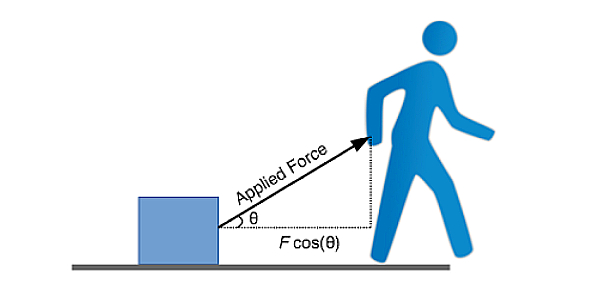# Energy Quiz To Challenge Your Physics Skills

12 Questions | Total Attempts: 60SettingsIn physics, energy is a quantitative force that must be transferred from one object to another, and to perform any kind of work on, or to change the state of the object. Energy is known to be a conserved quantity; the law of conservation states that energy can be transferred from one form to another, but not created or destroyed. Want to know about different types of energy? Take this quiz to find out about different types of energies.

• 1.
The transfer of thermal energy is ...
• A.

Heat

• B.

Energy

• C.

Work

• D.

Movement

• 2.
What happens to the temperature of a object as it moves faster?
• A.

Temperature increases

• B.

Temperature decreases

• C.

Temperature stays the same

• 3.
What type of energy do plants use to make sugar in the photosynthetic process?
• A.

Light

• B.

Heat

• C.

Green

• D.

Kinetic

• 4.
What type of energy is stored in the particles of matter?
• A.

Chemical energy

• B.

Thermal energy

• C.

Kinetic energy

• 5.
What type of mechanical energy is due to the position of an object?
• A.

Potential energy

• B.

Kinetic energy

• C.

Thermal energy

• D.

Crazy energy

• 6.
The energy caused by an object's vibrations
• A.

Sound energy

• B.

Thermal energy

• C.

Kinetic energy

• D.

Kinetic energy

• 7.
Name the energy that is contained in the food?
• A.

Chemical energy

• B.

Kinetic energy

• C.

Thermal energy

• 8.
What kind of energy does person at the top of the hill have after the ball has been pushed?
• A.

Potential energy

• B.

Kinetic energy

• C.

Thermal energy

• D.

Moving energy

• 9.
Energy is the ability to do ______. The answer is in this scrambled word -    rkow
• 10.
Which statement best summarizes the Principle of the Conservation of Energy?
• A.

Total energy is constant

• B.

Some energy is lost

• C.

Total energy can change

• D.

Energy is the ability to get stuff done

• 11.
Name the types of energy that are stored and are waiting to be used?
• A.

Chemical

• B.

Electical

• C.

Elastic

• D.

Gravitational potential

• E.

Light

• 12.
What type of energy is shown in the picture
• A.

Elastic

• B.

Potential

• C.

Kinetic

• D.

Chemical

• E.

Nuclear

• F.

Mechanical

Related TopicsBack to top